# Binary to BCD Code Converter

BCD is binary coded decimal number, where each digit of a decimal number is respected by its equivalent binary number. That means, LSB of a decimal number is represented by its equivalent binary number and similarly other higher significant bits of decimal number are also represented by their equivalent binary numbers.

For example, BCD Code of 14 is-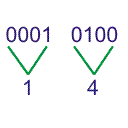Let us design a 4bit binary to BCD code converter. As the 4 bit can represent 0 to 15, we can draw the conversion table as follows,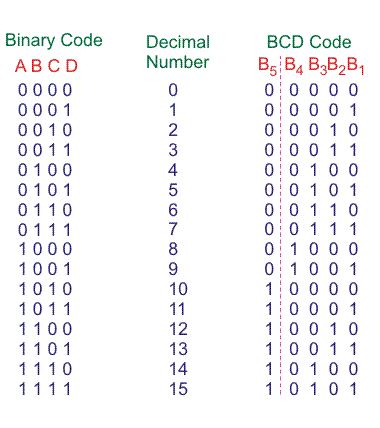Here, B5 bit represents MSB of decimal number and B4, B3, B2, B1 represents 4 bit binary equivalent of LSB of decimal number.

From, above conversion table, we can write SOP form for different bits of BCD code.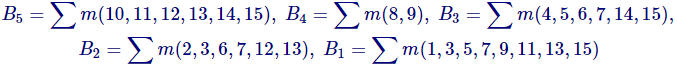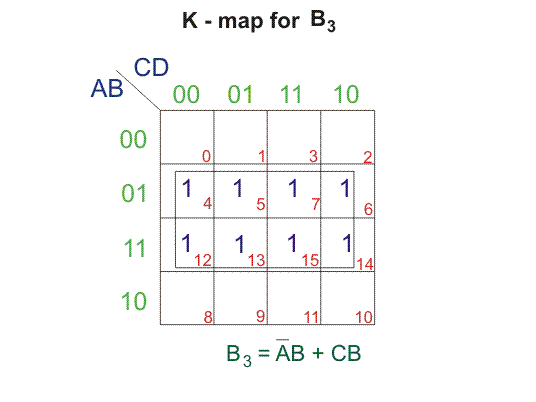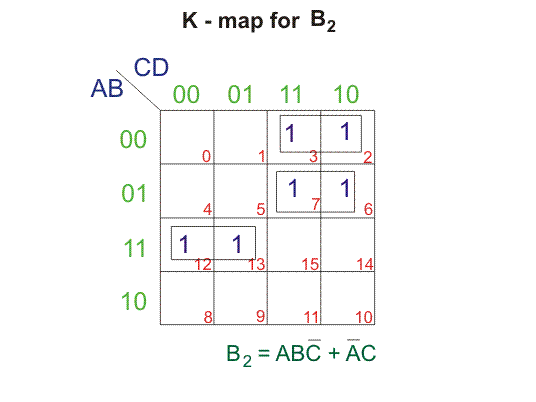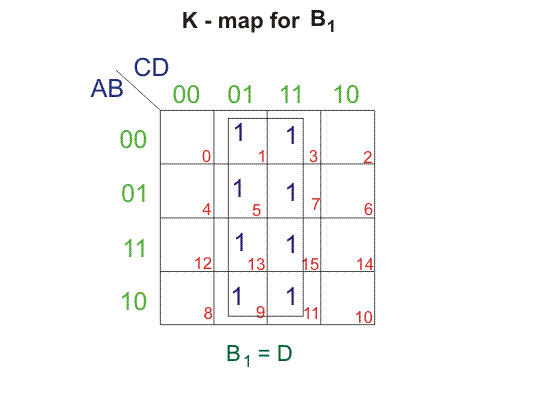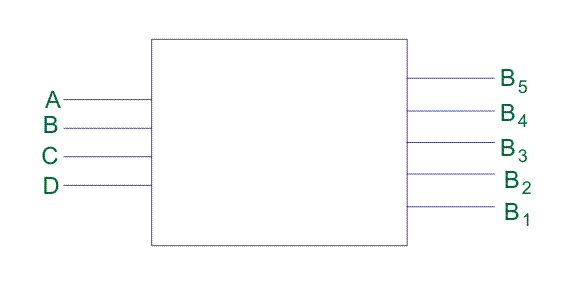Want To Learn Faster? 🎓
Get electrical articles delivered to your inbox every week.
No credit card required—it’s 100% free.